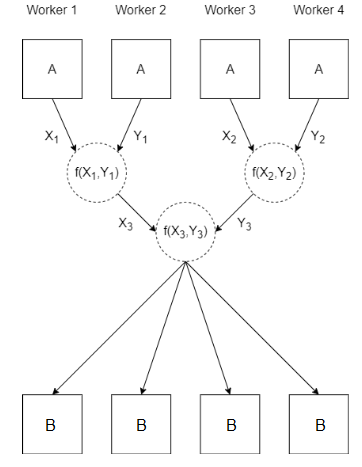# spmdReduce

Reduce arrays on `spmd` workers

Since R2022b

## Syntax

``B = spmdReduce(fcn,A)``
``B = spmdReduce(fcn,A,destination)``

## Description

example

````B = spmdReduce(fcn,A)` uses the function `fcn` to reduce the array `A` defined on each worker running an `spmd` block or communicating job. The function stores the result B of reduction on every worker.For example, `spmdReduce(@plus,A)` returns the sum of the arrays `A` defined on each worker.MATLAB® uses the `fcn` function to reduce AJ by calling the function N - 1 times. N is the number of workers running the `spmd` block or communicating job. To get the number of workers running the current spmd block, use the `spmdSize` functionAj is the array `A` defined on `spmd` worker whose index is j.To ensure that your `spmd` block or communicating job always produces the same results, specify `fcn` as an associative function.When you use `parfor`, `parfeval`, or `parfevalOnAll` to run code on a parallel pool, the workers are independent and do not communicate with each other. If you use `spmdReduce` on these workers, the result is the same as using `spmdReduce` on a client.If one worker is running the current spmd block, `B` is equal to `A`.```
````B = spmdReduce(fcn,A,destination)` reduces `A` and stores the result on only one worker.```

## Examples

collapse all

This example shows how to use `spmdReduce` to calculate the maximum value of an array across all workers.

Create a parallel pool with four workers.

`parpool(4);`

When you execute an `spmd` block after creating a parallel pool, by default all available workers in the pool run the code inside the `spmd` block.

Run `spmdIndex` on each worker in the `spmd` block and store the result in `A`. Use `spmdReduce` and `max` to calculate the maximum value of `A` from each worker.

Unless you specify a destination, `spmdReduce` stores the result on every worker. On the client, the results is a `Composite` object. To get the result, index into the `Composite` object.

```spmd A = spmdIndex; B = spmdReduce(@max,A); end disp(B{1})```
` 4`

## Input Arguments

collapse all

Input array, specified as a scalar, vector, matrix, multidimensional array, table, timetable, or any MATLAB variable that supports concatenation.

Example: `A = magic(3)`

Reduction function, specified as a function handle. The reduction function must take two input arguments.

Example: `fcn = @max`

Data Types: `function_handle`

Index of the destination worker, specified as a positive integer or an empty array. The value of this input must be less than or equal to the number of workers running the current `spmd` block or communicating job.

When you specify this input, the function stores the value of `B` only on the worker with this index. On the worker whose index is equal to `destination`, `B` is the result of the operation. On all other workers, `B` is `[]`.

Example: `1`

## Algorithms

This figure shows how the `spmdReduce` function uses `fcn` when you call `spmdReduce(fcn,A)`.## Version History

Introduced in R2022b